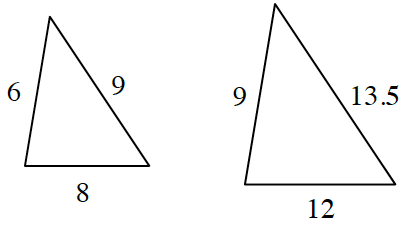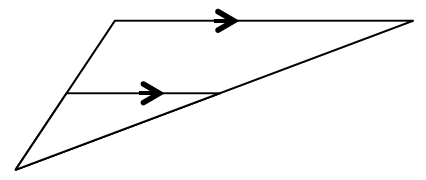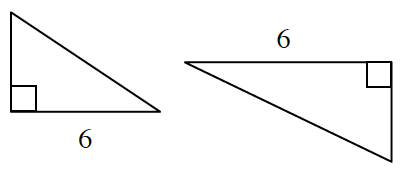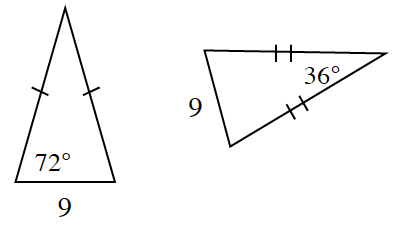### Home > GC > Chapter 7 > Lesson 7.1.3 > Problem7-28

7-28.

Recall the three similarity shortcuts for triangles: $\text{SSS}\sim$, $\text{SAS}\sim$, and $\text{AA}\sim$. For each pair of triangles below, decide whether the triangles are congruent and/or similar. Justify each conclusion.

1.Compare the ratios of each of the pairs of corresponding sides of the two triangles.

1.The two triangles are similar. Justify this by examining the angles of each triangle.

1.There is not enough information to determine whether or not the two triangles are similar.

1.Both triangles are isosceles. Use this information and the Triangle Sum Theorem to find the values of the unknown angles.